UPSC  >  Test: Permutation & Combination- 3

# Test: Permutation & Combination- 3

Test Description

## 15 Questions MCQ Test UPSC Prelims Paper 2 CSAT - Quant, Verbal & Decision Making | Test: Permutation & Combination- 3

Test: Permutation & Combination- 3 for UPSC 2023 is part of UPSC Prelims Paper 2 CSAT - Quant, Verbal & Decision Making preparation. The Test: Permutation & Combination- 3 questions and answers have been prepared according to the UPSC exam syllabus.The Test: Permutation & Combination- 3 MCQs are made for UPSC 2023 Exam. Find important definitions, questions, notes, meanings, examples, exercises, MCQs and online tests for Test: Permutation & Combination- 3 below.
Solutions of Test: Permutation & Combination- 3 questions in English are available as part of our UPSC Prelims Paper 2 CSAT - Quant, Verbal & Decision Making for UPSC & Test: Permutation & Combination- 3 solutions in Hindi for UPSC Prelims Paper 2 CSAT - Quant, Verbal & Decision Making course. Download more important topics, notes, lectures and mock test series for UPSC Exam by signing up for free. Attempt Test: Permutation & Combination- 3 | 15 questions in 30 minutes | Mock test for UPSC preparation | Free important questions MCQ to study UPSC Prelims Paper 2 CSAT - Quant, Verbal & Decision Making for UPSC Exam | Download free PDF with solutions
 1 Crore+ students have signed up on EduRev. Have you?
Test: Permutation & Combination- 3 - Question 1

### A gambler began playing blackjack with \$110 in chips. After exactly 12 hands, he left the table with \$320 in chips, aving won some hands and lost others. Each win earned \$100 and each loss cost \$10. How many possible  utcomes were there for the first 5 hands he played? (For example, won the first hand, lost the second, etc.)

Detailed Solution for Test: Permutation & Combination- 3 - Question 1

Let W be the number of wins and L be the number of losses. Since the total number of hands equals 12 and the net winnings equal \$210, we can construct and solve the following simultaneous equations: w + l = 12, 100 w – 10 l = 210. so l = 9, w = 3. So we know that the gambler won 3 hands and lost 9. We do not know where in the sequence of 12 hands the 3 wins appear. So when counting the possible outcomes for the first 5 hands, we must consider these possible scenarios: 1) Three wins and two losses 2) Two wins and three losses 3) One win and four losses 4) No wins and five losses In the first scenario, we have WWWLL. We need to know in how many different ways we can arrange these five letters: 5!/2!3! = 10. So there are 10 possible arrangements of 3 wins and 2 losses. The second scenario --WWLLL -- will yield the same result: 10. The third scenario -- WLLLL -- will yield 5 possible arrangements, since the one win has only 5 possible positions in the sequence. The fourth scenario --LLLLL -- will yield only 1 possible arrangement, since rearranging these letters always yields the same sequence. Altogether, then, there are 10 + 10 + 5 + 1 = 26 possible outcomes for the gambler's first five hands.

Test: Permutation & Combination- 3 - Question 2

### In a 4 person race, medals are awarded to the fastest 3 runners. The first-place runner receives a gold medal, the second-place runner receives a silver medal, and the third-place runner receives a bronze medal. In the event of a tie, the tied runners receive the same color medal. (For example, if there is a two-way tie for firstplace, the top two runners receive gold medals, the next-fastest runner receives a silver medal, and no bronze medal is awarded). Assuming that exactly three medals are awarded, and that the three medal winners stand together with their medals to form a victory circle, how many different victory circles are possible?

Detailed Solution for Test: Permutation & Combination- 3 - Question 2

First, let's consider the different medal combinations that can be awarded to the 3 winners: (1) If there are NO TIES then the three medals awarded are: GOLD, SILVER, BRONZE. (2) What if there is a 2-WAY tie? --If there is a 2-WAY tie for FIRST, then the medals awarded are: GOLD, GOLD, SILVER. --If there is a 2-WAY tie for SECOND, then the medals awarded are: GOLD, SILVER, SILVER. --There cannot be a 2-WAY tie for THIRD (because exactly three medals are awarded in total). (3) What if there is a 3-WAY tie? --If there is a 3-WAY tie for FIRST, then the medals awarded are: GOLD, GOLD, GOLD. --There are no other possible 3-WAY ties. Thus, there are 4 possible medal combinations: (1) G, S, B (2) G, G, S (3) G, S, S (4) G, G, G. Now let's determine how many different ways each combination can be distributed. We'll do this by considering four runners: Albert, Bob, Cami, and Dora.
COMBINATION 1: Gold, Silver, Bronze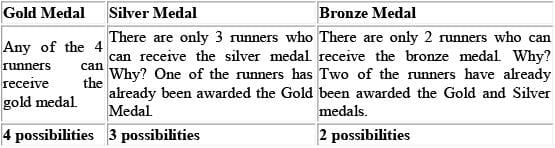Therefore, there are different victory circles that will contain 1 GOLD, 1 SILVER, and 1 BRONZE medalist. COMBINATION 2: Gold, Gold, Silver.
Using the same reasoning as for Combination 1, we see that there are 24 different victory circles that will contain 2 GOLD medalists and 1 SILVER medalist. However, it is important to realize that these 24 victory circles must be reduced due to "overcounting." To illustrate this, consider one of the 24 possible Gold-GoldSilver victory circles: Albert is awarded a GOLD. Bob is awarded a GOLD. Cami is awarded a SILVER. Notice that this is the exact same victory circle as the following: Bob is awarded a GOLD. Albert is awarded a GOLD. Cami is awarded a SILVER. Each victory circle has been "overcounted" because we have been counting each different ordering of the two gold medals as a unique victory circle, when, in reality, the two different orderings consist of the exact same victory circle. Thus, the 24 victory circles must be cut in half; there are actually only 12 unique victory circles that will contain 2 GOLD medalists and 1 SILVER medalist. (Note that we did not have to worry about "overcounting" in Combinat ion 1, because each of those 24 possibilit ies was unique.)
COMBINATION 3: Gold, Silver, Silver.
Using the same reasoning as for Combination 2, we see that there are 24 possible victory circles, but only 12 unique victory circles that contain 1 GOLD medalist and 2 SILVER medalists.
COMBINATION 4: Gold, Gold, Gold.
Here, once again, there are 24 possible victory circles. However, because all three winners are gold medalists, there has been a lot of "overcounting!" How much overcounting? Let's consider one of the 24 possible GoldGold-Gold victory circles: Albert is awarded a GOLD. Bob is awarded a GOLD. Cami is awarded a GOLD.
Notice that this victory circle is exact ly the same as the following victory circles: Albert-GOLD, Cami-GOLD, Bob-GOLD. Bob-GOLD, Albert-GOLD, Cami-GOLD. Bob-GOLD, Cami-GOLD, Albert-GOLD. CamiGOLD, Albert-GOLD, Bob-GOLD. Cami-GOLD, Bob-GOLD, Albert-GOLD. Each unique victory circle has actually been counted 6 times! Thus we must divide 24 by 6 to find the number of unique victory circles. There are actually only unique victory circles that contain 3 GOLD medalists. FINALLY, then, we have the following:
(Combination 1) 24 unique GOLD-SILVER-BRONZE victory circles. (Combination 2) 12 unique GOLDGOLD-SILVER victory circles. (Combination 3) 12 unique GOLD-SILVER-SILVER victory circles. (Combination 4) 4 unique GOLD-GOLD-GOLD victory circles. Thus, there are unique victory circles.

Test: Permutation & Combination- 3 - Question 3

### The organizers of a week-l ong fair have hired exactly five security guards to patrol the fairgrounds at night for the duration of the event. Exactly two guards are assigned to patrol the grounds every night, with no guard assigned consecutive nights. If the fair begins on a Monday, how many different pairs of guards will be available to patrol the fairgrounds on the following Saturday night?

Detailed Solution for Test: Permutation & Combination- 3 - Question 3

This question is not as complicated as it may initially seem. The trick is to recognize a recurring pattern in the assignment of the guards. First, we have five guards (let's call them a, b, c, d, and e) and we have to break them down into pairs. So how many pairs are possible in a group of five dist inct ent ities? We could use the combinations formula: nCr. where n is the number of items you are selecting from (the pool) and k is the number of items you are selecting (the subgroup). Here we would get 5C2 = 10. So there are 10 different pairs in a group of 5 individuals. Ho wever, in this particular case, it is actually more helpful to write them out (since there are only 5 guards and 10 pairs, it is not so onerous): ab, ac, ad, ae, bc, bd, be, cd, ce, de. Now, on the first night (Monday), any one of the ten pairs may be assigned, since no one has worked yet. Let's say that pair ab is assigned to work the first night. That means no pair containing either a or b may be assigned on Tuesday night. That rules out 7 of the 10 pairs, leaving only cd, ce, and de available for assignment. If, say, cd were assigned on Tuesday, then on Wednesday no pair containing either c or d could be assigned. This leaves only 3 pairs available for Wednesday: ab, ae, and be. At this point the savvy test taker will realize that on any given night after the first, including Saturday, only 3 pairs will be available for assignment. Those test takers who are really on the ball may have realized right away that the assignment of any two guards on any night necessarily rules out 7 of the 10 pairs for the next night, leaving only 3 pairs available on all nights after Monday. The correct answer is Choice D; 3 different pairs will be available to patrol the grounds on Saturday night.

Test: Permutation & Combination- 3 - Question 4

Larry, Michael, and Doug have five donuts to share. If any one of the men can be given any whole number of donuts from 0 to 5, in how many different ways can the donuts be distributed?

Detailed Solution for Test: Permutation & Combination- 3 - Question 4

The key to this problem is to avoid list ing all the possibilit ies. Instead, think of an arrangement of five donuts and two dividers. The placement of the dividers determines which man is allotted which donuts, as pictured below:In this example, the first man receives one donut, the second man receives three donuts, and the third man receives one donut. Remember that it is possible for either one or two of the men to be allotted no donuts at all. This situation would be modeled with the arrangement below:Here, the second man receives no donuts. Now all that remains is to calculate the number of ways in which the donuts and dividers can be arranged: There are 7 objects. The number of ways in which 7 objects can be arranged can be computed by taking 7!: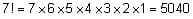However, the two dividers are identical, and the five donuts are identical. Therefore, we must divide 7! by 2! and by 5!: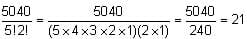Test: Permutation & Combination- 3 - Question 5

A woman has seven cookies—four chocolate chip and three oatmeal. She gives one cookie to each of her six children: Nicole, Ronit, Kim, Deborah, Mark, and Terrance. If Deborah will only eat the kind of cookie that Kim eats, in how many different ways can the cookies be distributed? (The leftover cookie will be given to the dog.)

Detailed Solution for Test: Permutation & Combination- 3 - Question 5

Test: Permutation & Combination- 3 - Question 6

How many different combinations of outcomes can you make by rolling three standard (6-sided) dice if the order of the dice does not matter?

Detailed Solution for Test: Permutation & Combination- 3 - Question 6

The three-dice combinations fall into 3 categories of outcomes: 1) All three dice have the same number 2) Two of the dice have the same number with the third having a different number than the pair 3) All three dice have different numbers By calculating the number of combinations in each category, we can determine the total number of different possible outcomes by summing the number of possible outcomes in each category.
First, let’s calculate how many combinations can be made if all 3 dice have the same number. Since there are only 6 numbers, there are only 6 ways for all three dice to have the same number (i.e., all 1’s, or all 2’s, etc.). Second, we can determine how many combinations can occur if only 2 of the dice have the same number. There are 6 different ways that 2 dice can be paired (i.e., two 1’s, or two 2’s or two 3’s, etc.). For each given pair of 2 dice, the third die can be one of the five other numbers. (For example if two of the dice are 1’s, then the third die must have one of the 5 other numbers: 2, 3, 4, 5, or 6.) Therefore there are 6 x 5 = 30 combinations of outcomes that involve 2 dice with the same number. Third, determine how many combinations can occur if all three dice have different numbers. Think of  choosing three of the 6 items (each of the numbers) to fill three "slots." For the first slot, we can choose from 6 possible items. For the second slot, we can choose from the 5 remaining items. For the third slot, we can choose from the 4 remaining items. Hence, there are 6 x 5 x 4 = 120 ways to fill the three slots. However, we do not care about the order of the items, so permutations like {1,2,5}, {5, 2, 1}, {2, 5, 1}, {2, 1, 5}, {5, 1, 2}, and {1, 5, 2} are all considered to be the same result. There are 3! = 6 ways that each group of three numbers can be ordered, so we must divide 120 by 6 in order to obtain the number of combinations where order does not matter (every 1 combination has 6 equivalent permutations). Thus there are combinations where all three dice have different numbers. The total number of combinations is the sum of those in each category or 6 + 30 + 20 = 56.

Test: Permutation & Combination- 3 - Question 7

A certain league has four divisions. The respective divisions had 9, 10, 11, and 12 teams qualify for the playoffs. Each division held its own double-elimination tournament -- where a team is eliminated from the tournament upon losing two games -- in order to determine its champion. The four division champions then played in a single-elimination tournament -- where a team is eliminated upon losing one game -- in order to determine the overall league champion. Assuming that there were no ties and no forfeits, what is the maximum number of games that could have been played in order to determine the overall league champion?

Detailed Solution for Test: Permutation & Combination- 3 - Question 7

There are two different approaches to solving this problem. The first employs a purely algebraic approach, as follows: Let us assume there are n teams in a double-eliminat ion tournament. In order to crown a champion, n – 1 teams must be eliminated, each losing exactly two games. Thus, the minimum number of games played in order to eliminate all but one of the teams is 2(n – 1). At the time when the (n – 1)th team is eliminated, the surviving team (the division champion) either has one loss or no losses, adding at most one more game to the total played. Thus, the maximum number of games that can be played in an n-team double-eliminat ion tournament is 2(n – 1) + 1. There were four divisions with 9, 10, 11, and 12 teams each. The maximum number of games that could have been played in order to determine the four division champions was (2(8) + 1) + (2(9) + 1) + (2(10) + 1) + (2(11) + 1) = 17 + 19 + 21 + 23 = 80. The four division champions then played in a single-elimination tournament. Since each team that was eliminated lost exactly one game, the elimination of three teams required exactly three more games. Thus, the maximum number of games that could have been played in order to crown a league champion was 80 + 3 = 83. The correct answer choice is (B). Another way to approach this problem is to use one division as a concrete starting point. Let's think first about the 9-team division. After 9 games, there are 9 losses. Assuming that no team loses twice (thereby maximizing the number of games played), all 9 teams remain in the tournament. After 8 additional games, only 1 team remains and is declared the division winner. Therefore, 9 + 8 = 17 games is the maximum # o f games than can be played in this tournament. We can generalize this information and apply it to the other divisions. To maximize the # of games in the 10-team division, 10 + 9 = 19 games are played. To maximize the # of games in the 11-team division, 11 + 10 = 21 games are played. To maximize the # of games in the 12-team division, 12 + 11 = 23 games are played. Thus, the maximum number of games that could have been played in order to determine the four division champions was 17 + 19 + 21 + 23 = 80. After 3 games in the single elimination tournament, there will be 3 losses, thereby eliminating all but the one championship team. Thus, the maximum number of games that could have been played in order to crown a league champion was 80 + 3 = 83. Once again.

Test: Permutation & Combination- 3 - Question 8

You have a bag of 9 letters: 3 Xs, 3 Ys, and 3 Zs. You are given a box divided into 3 rows and 3 columns for a total of 9 areas. How many different ways can you place one letter into each area such that there are no rows or columns with 2 or more of the same letter?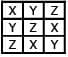Detailed Solution for Test: Permutation & Combination- 3 - Question 8

The simplest way to solve this problem is to analyze one row at a time, and one square at a time in each row if necessary. Let’s begin with the top row. First, let’s place a letter in left box; we have a choice of 3 different letters for this box: X, Y, or Z. Next, we place a letter in the top center box. Now we have only 2 options so as not to match the letter we placed in the left box. Finally, we only have 1 letter to choose for the right box so as not to match eit her of the letters in the first two boxes. Thus, we have 3 x 2 x 1 or 6 ways to fill in the top row without duplicating a letter across it. Now let’s analyze the middle row by assuming that we already have a particular arrangement of the top row, say the one given in the example above (XYZ).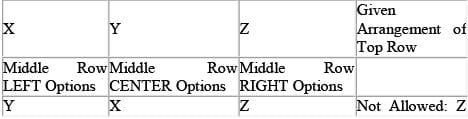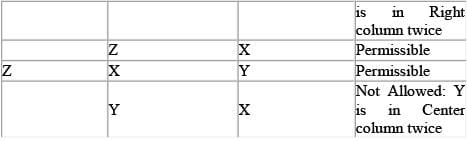Now let’s analyze the bottom row by assuming that we already have a particular arrangement of the top and middle rows. Again, let’s use top and middle row arrangements given in the example above.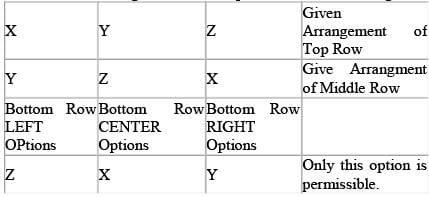We can see that given fixed top and middle rows, there is only 1 possible bottom row that will work. (In other words, the 3rd row is completely determined by the arrangement of the 1st and 2nd rows). By combining the informat ion about each row, we calculate the solut ion as follows: 6 possible top rows × 2 possible middle rows × 1 possible bottom row = 12 possible grids.

Test: Permutation & Combination- 3 - Question 9

Eight women of eight different heights are to pose for a photo in two rows of four. Each woman in the second row must stand directly behind a shorter woman in the first row. In addition, all of the women in each row must be arranged in order of increasing height from left to right. Assuming that these restrictions are fully adhered to, in how many different ways can the women pose?

Detailed Solution for Test: Permutation & Combination- 3 - Question 9

This is a counting problem that is best solved using logic. First, let’s represent the line of women as follows:
0000
0000
where the heights go from 1 to 8 in increasing order and the unknowns are designated 0s. Since the women are arranged by their heights in increasing order from left to right and front to back, we know that at a minimum, the lineup must conform to this:
0008
1000
Let’s further designate the arrangement by labeling the other individuals in the top row as X, Y and Z, and the individuals in the bottom row as A, B, and C.
XYZ8
1ABC
Note that Z must be greater than at least 5 numbers (X, Y, B, A, and 1) and less than at least 1 number (8). This means that Z can only be a 6 or a 7. Note that Y must be greater than at least 3 numbers (X, A and 1) and less than at least 2 numbers (8 and Z). This means that Y can only be 4, 5, or 6. Note that X must be greater than at least 1 number (1) and less than at least 3 numbers (8, Z and Y), This means that X must be 2, 3, 4, or 5. This is enough information to start counting the total number of possibilities for the top row. It will be easiest to use the middle unknown value Y as our starting point. As we determined above, Y can only be 4, 5, or 6. Let’s check each case, making our conclusions logically: If Y is 4, Z has 2 options (6 or 7) and X has 2 options (2 or 3). This yields 2 x 2 = 4 possibilities. If Y is 5, Z has 2 options (6 or 7), and X has 3 options (2, 3, or 4). This yields 2 x 3 = 6 possibilities. If Y is 6, Z has 1 option (7), and X has 4 options (2, 3, 4, or 5). This yields 1 x 4 = 4 possibilities. For each of the possibilities above, the bottom row is completely determined because we have 3 numbers left, all of
which must be in placed increasing order. Hence, there are 4 + 6 + 4 = 14 ways for the women to pose.

Test: Permutation & Combination- 3 - Question 10

You have a six-sided cube and six cans of paint, each a different color. You may not mix colors of paint. How many distinct ways can you paint the cube using a different color for each side? (If you can reorient a cube to look like another cube, then the two cubes are not distinct.)

Detailed Solution for Test: Permutation & Combination- 3 - Question 10

This is a relat ively simple problem that can be fiendishly difficult unless you have a good approach to solving it and a solid understanding of how to count. We will present two different strategies here. Strategy 1: This problem seems difficult, because you need to figure out how many distinct orientations the cube has relative to its other sides. Given that you can rotate the cube in an unlimited number of ways, it is very difficult to keep track of what is going on – unless you have a system. Big hint: In order to analyze how mult iple things behave or compare or are arranged relative to each other, the first thing one should do is pick a reference point and fix it. Here is a simple example. Let’s say you have a round table with four seat positions and you want to know how many distinct ways you can orient 4 people around it relative to each other (i.e., any two orientations where all 4 people have the same person to their left and to their right are considered equivalent). Let’s pick person A as our reference po int and anchor her to the North position. Think about this next statement and convince yourself that it is true: By choosing A as a fixed reference, all distinct arrangements of the other 3 people relative to A will constitute the complete set of distinct arrangements of all 4 people relative to each other. Hence, fixing the location of one person makes it significantly easier to keep track of what is going on. Given A is fixed at the North, the 3 other people can be arranged in the 3 remaining seats in 3! = 6 ways, so there are 6 dist inct orientations of 4 people sitt ing around a circular table. Using the same principle, we can conclude that, in general, if there are N people in a circular arrangement, after fixing one person at a reference point, we have (N-1)! distinct arrangements relative to each other. Now let’s solve the problem. Assume the six sides are: Top (or T), Bottom (or B), N, S, E, and W, and the six colors are designated 1, 2, 3, 4, 5, and 6. Following the first strategy, let’s pick color #1 and fix it on the Top side of the cube. If #1 is at the Top position, then one of the other 5 colors must be at the Bottom position and each of those colors would represent a distinct set of arrangements. Hence, since there are exactly 5 possible choices for the color of the Bottom side, the number of unique arrangements relative to #1 in the Top position is a multiple of 5. For each of the 5 colors paired with #1, we need to arrange the other 4 colors in the N, S, E, and W positions in distinct arrangements. Well, this is exactly like arranging 4 people around a circular table, and we have already determined that there are (n-1)! or 3! ways to do that. Hence, the number of distinct patterns of painting the cube is simply 5 x 3! = 30.
Strategy 2: There is another way to solve this kind of problem. Given one dist inct arrangement or pattern, you can try to determine how many equivalent ways there are to represent that one particular arrangement or pattern within the set of total permutations, then divide the total number of permutations by that number to get the number of distinct arrangements. This is best illustrated by example so let’s go back to the 4 people arranged around a circular table. Assume A is in the North posit ion, then going clockwise we get B, then C, then D. Rotate the table 1/4 turn clockwise. Now we have a different arrangement where D is at the North position, followed clockwise by A, then B, then C. BUT, this is merely a rotation of the distinct relative position of the 4 people (i.e., everyone still has the same person to his right and to his left) so they are actually the same arrangement. We can quickly conclude that there are 4 equivalent or non-dist inct arrangements for every dist inct relat ive posit ioning of the 4 people. We can arrange 4 people in a total of 4! = 24 ways. However, each DISTINCT arrangement has 4 equivalents, so in order to find the number of distinct arrangements, we need to divide 4! by 4, which yields 3! or 6 distinct ways to arrange 4 people around a circular table, the same result we got using the “fixed reference” method in Strategy 1. Generalizing, if there are N! ways to arrange N people around a table, each dist inct relat ive rotation can be represented in N ways (each 1/Nth rotation around the table) so the number of dist inct arrangements is N!/N = (N-1)! Now let’s use Strategy #2. Consider a cube that is already painted in a particular way. Imagine putting the cube on the table, with color #1 on the top side. Note, that by rotating the cube, we have 4 different orientations of this particular cube given color #1 is on top. Using symmetry, we can repeat this analysis when #1 is facing any of the other 5 directions. Hence, for each of the six directions that the side painted with #1 can face, there are 4 ways to orient the cube. Consequently, there are 6 x 4 = 24 total orientations of any one cube painted in a particular manner. Since there are 6 sides and 6 colors, there are 6! or 720 ways to color the six sides each with one color. However, we have just calculated that each DISTINCT pattern has 24 equivalent orientations, so 720 must be divided by 24 to get the number of distinct patterns. This yields 720/24 = 30, confirming the answer found using Strategy #1.

Test: Permutation & Combination- 3 - Question 11

A group of four women and three men have tickets for seven adjacent seats in one row of a theatre. If the three men will not sit in three adjacent seats, how many possible different seating arrangements are there for these 7 theatre-goers?

Detailed Solution for Test: Permutation & Combination- 3 - Question 11

The first thing to recognize here is that this is a permutation with restrictions quest ion. In such questions it is always easiest to tackle the restricted scenario(s) first. The restricted case here is when all of the men actually sit together in three adjacent seats. Restrict ions can often be dealt with by considering the limited individuals as one unit. In this case we have four women ( w1, w2, w3, and w4) and three men (m1, m2, and m3). We can consider the men as one unit, since we can think of the 3 adjacent seats as simply 1 seat. If the men are one unit (m), we are really looking at seating 5 individuals ( w1, w2, w3, w4, and m) in 5 seats. There are 5! ways of arranging 5 individuals in a row. This means that our group of three men is sitting in any of the “five” seats. Now, imagine that the one seat that holds the three men magically splits into three seats. How many different ways can the men arrange themselves in those three seats? 3!. To calculate the total number of ways that the men and women can be arranged in 7 seats such that the men ARE sitting together, we must multiply these two values: 5!3!. However this problem asks for the number of ways the theatre-goers can be seated such that the men are NOT seated three in a row. Logically, this must be equivalent to the following: (Total number of all seat arrangements) – (Number of arrangements with 3 men in a row). The total number of all seat arrangements is simply 7! so the final calculat ion is 7! – 5!3!.

Test: Permutation & Combination- 3 - Question 12

Anthony and Michael sit on the six-member board of directors for company X. If the board is to be split up into 2 three-person subcommittees, what percent of all the possible subcommittees that include Michael also include Anthony?

Detailed Solution for Test: Permutation & Combination- 3 - Question 12

It is important to first note that our point of reference in this quest ion is all the possible subco mmittees that include Michael. We are asked to find what percent of these subcommittees also include Anthony. Let's first find out how many possible subcommittees there are that must include Michael. If Michael must be on each of the three-person committees that we are considering, we are essentially choosing people to fill the two remaining spots of the committee. Therefore, the number of possible committees  can be found by considering the number of different two-people groups that can be formed from a pool of 5 candidates (not 6 since Michael was already chosen). Using the anagram method to solve this combinations question, we assign 5 letters to the various board members in the first row. In the second row, two of the board members get assigned a Y to signify that they were chosen and the remaining 3 get an N, to signify that they were not chosen: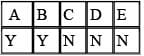The number of different combinations of two-person committees from a group of 5 board members would be the number of possible anagrams that could be formed from the word YYNNN = 5! / (3!2!) = 10. Therefore there are 10 possible committees that include Michael. Out of these 10 possible committees, of how many will Anthony also be a member? If we assume that Anthony and Michael must be a member of the three-person committee, there is only one remaining place to fill. Since there are four other board members, there are four possible three-person committees with both Anthony and Michael. Of the 10 committees that include Michael, 4/10 or 40% also include Anthony. The correct answer is C. As an alternate method, imagine splitting the original six-person board into two equal groups of three. Michael is automatically in one of those groups of three. Now, Anthony could occupy any one of the other 5 posit ions -- the 2 on Michael's committee and the 3 on the other committee. Since Anthony has an equal chance of winding up in any of those positions, his chance of landing on Michael's committee is 2 out of 5, or 2/5 = 40%. Since that probabilit y must correspond to the ratio of commit tees asked for in the problem, the answer is achieved.

Test: Permutation & Combination- 3 - Question 13

A family consisting of one mother, one father, two daughters and a son is taking a road trip in a sedan. The sedan has two front seats and three back seats. If one of the parents must drive and the two daughters refuse to sit next to each other, how many possible seating arrangements are there?

Detailed Solution for Test: Permutation & Combination- 3 - Question 13

The easiest way to solve this quest ion is to consider the restrictions separately. Let’s start by considering the restriction that one of the parents must drive, temporarily ignoring the restriction that the two sisters won't sit next to each other. This means that…
2 people (mother or father) could sit in the driver’s seat 4 people (remaining parent or one of the children) could sit in the front passenger seat 3 people 0could sit in the first back seat 2 people could sit in the second back seat 1 person could sit in the remaining back seat.
The total number of possible seat ing arrangements would be the product of these various possibilit ies: 2 × 4 × 3 × 2 × 1 = 48. We must subtract from these 48 possible seating arrangements the number of seating arrangements in which the daughters are sitting together. The only way for the daughters to sit next to each other is if they are both sitting in the back. This means that…  2 people (mother or father) could sit in the driver’s seat 2 people (remaining parent or son) could sit in the front passenger seat Now for the back three seats we will do something a little different. The back three seats must contain the two daughters and the remaining person (son or parent). To find out the number of arrangements in which the daughters are sitting adjacent, let’s consider the two daughters as one unit. The remaining person (son or parent) is the other unit. Now, instead of three seats to fill, we only have two "seats," or units, to fill.
There are 2 × 1 = 2 ways to seat these two units. However, the daughter-daughter unit could be d1d2 or d2d1 We must consider both of these possibilities so we multiply the 2 by 2! for a total of 4 seating possibilities in the back. We could also have manually counted these possibilities: d1d2X, d2d1X, Xd1d2, Xd2d1 Now we must multiply these 4 back seat scenarios by the front seat scenarios we calculated earlier:
(2 × 2) × 4 = 16 front back If we subtract these 16 "daughters-sitting-adjacent" scenarios from the total number of "parent-driving" scenarios, we get: 48 – 16 = 32

Test: Permutation & Combination- 3 - Question 14

Six mobsters have arrived at the theater for the premiere of the film “Goodbuddies.”  One of the mobsters, Frankie, is an informer, and he's afraid that another member of his crew, Joey, is on to him. Frankie, wanting to keep Joey in his sights, insists upon standing behind Joey in line at the concession stand.  How many ways can the six arrange themselves in line such that Frankie’s requirement is satisfied?

Detailed Solution for Test: Permutation & Combination- 3 - Question 14

Ignoring Frankie's requirement for a moment, observe that the six mobsters can be arranged 6! or 6 x 5 x 4 x 3 x 2 x 1 = 720 different ways in the concession stand line. In each of those 720 arrangements, Frankie must be either ahead of or behind Joey. Logically, since the combinat ions favor neither Frankie nor Joey, each would be behind the other in precisely half of the arrangements. Therefore, in order to satisfy Frankie's requirement, the six mobsters could be arranged in 720/2 = 360 different ways.

Test: Permutation & Combination- 3 - Question 15

A student committee that must consist of 5 members is to be formed from a pool of 8 candidates. How many different committees are possible?

Detailed Solution for Test: Permutation & Combination- 3 - Question 15

To find the total number of possible committees, we need to determine the number of different fiveperson groups that can be formed from a pool of 8 candidates. We will use the anagram method to solve this co mbinat ions quest ion. First, let's create an anagram grid and assign 8 letters in the first row, with each letter representing one of the candidates. In the second row, 5 of the candidates get assigned a Y to signify that they were chosen for a committee; the remaining 3 candidates get an N, to signify that they were not chosen: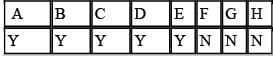The total number of possible five-person committees that can be created from a group of 8 candidates will be equal to the number of possible anagrams that can be formed from the word YYYYYNNN = 8! / (5!3!) = 56. Therefore, there are a total of 56 possible committees.

## UPSC Prelims Paper 2 CSAT - Quant, Verbal & Decision Making

67 videos|50 docs|151 tests
 Use Code STAYHOME200 and get INR 200 additional OFF Use Coupon Code
Information about Test: Permutation & Combination- 3 Page
In this test you can find the Exam questions for Test: Permutation & Combination- 3 solved & explained in the simplest way possible. Besides giving Questions and answers for Test: Permutation & Combination- 3, EduRev gives you an ample number of Online tests for practice

## UPSC Prelims Paper 2 CSAT - Quant, Verbal & Decision Making

67 videos|50 docs|151 tests

### How to Prepare for UPSC

Read our guide to prepare for UPSC which is created by Toppers & the best Teachers